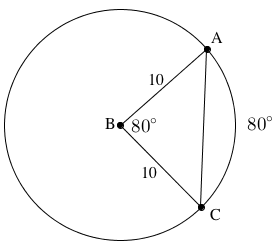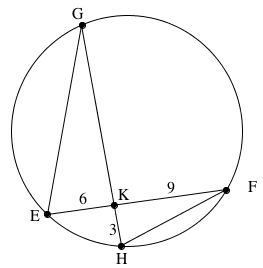### Home > CCG > Chapter Ch10 > Lesson 10.2.3 > Problem10-107

10-107.

Review circle relationships as you answer the questions below. Homework Help ✎

1. On your paper, draw a diagram of $⊙B$ with $\overarc{ A C }$. If $m\overarc{ A C }= 80º$ and the length of the radius of $⊙B$ is $10$, find the length of chord $\overline{ A C }$.

According to the Law of Cosines,
$b^2 = a^2 + c^2 − 2ab\cos(\text{B})$
Therefore, $b^2 = 10^2 + 10 − 2(10)(10)\cos(80º)$1. Now draw a diagram of a circle with two chords, $\overline{EF}$ and $\overline{GH}$, that intersect at point $K$. If $EF=15$, $EK=6$, and $HK=3$, what is $GK$?

Remember that intersecting chords create similar triangles, and that the corresponding sides of similar triangles have equal ratios.

$\frac{9}{3}=\frac{x}{6}$